x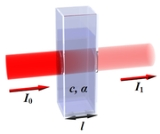Beer-Lambert lawOverview

In optics
Optics
Optics is the branch of physics which involves the behavior and properties of light, including its interactions with matter and the construction of instruments that use or detect it. Optics usually describes the behavior of visible, ultraviolet, and infrared light...

, the Beer–Lambert law, also known as Beer's law or the Lambert–Beer law or the Beer–Lambert–Bouguer law (named after August Beer
August Beer
August Beer was a German physicist and mathematician. Beer was born in Trier, where he studied mathematics and natural sciences. He worked for Julius Plücker in Bonn afterwards, where he earned a Ph.D. in 1848 and became a lecturer in 1850. In 1854, Beer published his book Einleitung in die höhere...

, Johann Heinrich Lambert
Johann Heinrich Lambert
Johann Heinrich Lambert was a Swiss mathematician, physicist, philosopher and astronomer.Asteroid 187 Lamberta was named in his honour.-Biography:...

, and Pierre Bouguer
Pierre Bouguer
Pierre Bouguer was a French mathematician, geophysicist, geodesist, and astronomer. He is also known as "the father of naval architecture"....

) relates the absorption
In physics, absorption of electromagnetic radiation is the way by which the energy of a photon is taken up by matter, typically the electrons of an atom. Thus, the electromagnetic energy is transformed to other forms of energy for example, to heat. The absorption of light during wave propagation is...

of light
Light
Light or visible light is electromagnetic radiation that is visible to the human eye, and is responsible for the sense of sight. Visible light has wavelength in a range from about 380 nanometres to about 740 nm, with a frequency range of about 405 THz to 790 THz...

to the properties of the material through which the light is travelling.
The law states that there is a logarithmic dependence between the transmission (or transmissivity), T, of light through a substance and the product of the absorption coefficient of the substance, α, and the distance the light travels through the material (i.e., the path length), ℓ.Is it just me, or are there no equations at all in this article?Encyclopedia
In optics
Optics
Optics is the branch of physics which involves the behavior and properties of light, including its interactions with matter and the construction of instruments that use or detect it. Optics usually describes the behavior of visible, ultraviolet, and infrared light...

, the Beer–Lambert law, also known as Beer's law or the Lambert–Beer law or the Beer–Lambert–Bouguer law (named after August Beer
August Beer
August Beer was a German physicist and mathematician. Beer was born in Trier, where he studied mathematics and natural sciences. He worked for Julius Plücker in Bonn afterwards, where he earned a Ph.D. in 1848 and became a lecturer in 1850. In 1854, Beer published his book Einleitung in die höhere...

, Johann Heinrich Lambert
Johann Heinrich Lambert
Johann Heinrich Lambert was a Swiss mathematician, physicist, philosopher and astronomer.Asteroid 187 Lamberta was named in his honour.-Biography:...

, and Pierre Bouguer
Pierre Bouguer
Pierre Bouguer was a French mathematician, geophysicist, geodesist, and astronomer. He is also known as "the father of naval architecture"....

) relates the absorption
In physics, absorption of electromagnetic radiation is the way by which the energy of a photon is taken up by matter, typically the electrons of an atom. Thus, the electromagnetic energy is transformed to other forms of energy for example, to heat. The absorption of light during wave propagation is...

of light
Light
Light or visible light is electromagnetic radiation that is visible to the human eye, and is responsible for the sense of sight. Visible light has wavelength in a range from about 380 nanometres to about 740 nm, with a frequency range of about 405 THz to 790 THz...

to the properties of the material through which the light is travelling.

## Equations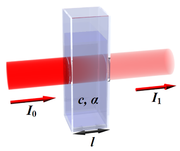The law states that there is a logarithmic dependence between the transmission (or transmissivity), T, of light through a substance and the product of the absorption coefficient of the substance, α, and the distance the light travels through the material (i.e., the path length), ℓ. The absorption coefficient can, in turn, be written as a product of either a molar absorptivity
Molar absorptivity
The molar absorption coefficient, molar extinction coefficient, or molar absorptivity, is a measurement of how strongly a chemical species absorbs light at a given wavelength...

(extinction coefficient) of the absorber, ε, and the concentration
Concentration
In chemistry, concentration is defined as the abundance of a constituent divided by the total volume of a mixture. Four types can be distinguished: mass concentration, molar concentration, number concentration, and volume concentration...

c of absorbing species in the material, or an absorption cross section
Absorption cross section
Absorption cross section is a measure for the probability of an absorption process. More generally, the term cross section is used in physics to quantify the probability of a certain particle-particle interaction, e.g., scattering, electromagnetic absorption, etc...

, σ, and the (number) density N' of absorbers.

For liquids, these relations are usually written as: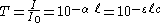whereas for gases, and in particular among physicists and for spectroscopy and spectrophotometry, they are normally written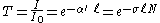where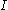0 andare the intensity
Intensity (physics)
In physics, intensity is a measure of the energy flux, averaged over the period of the wave. The word "intensity" here is not synonymous with "strength", "amplitude", or "level", as it sometimes is in colloquial speech...

(or power
Power (physics)
In physics, power is the rate at which energy is transferred, used, or transformed. For example, the rate at which a light bulb transforms electrical energy into heat and light is measured in watts—the more wattage, the more power, or equivalently the more electrical energy is used per unit...

) of the incident light and the transmitted light, respectively; σ is cross section of light absorption by a single particle and N is the density (number per unit volume) of absorbing particles.

The base 10
Common logarithm
The common logarithm is the logarithm with base 10. It is also known as the decadic logarithm, named after its base. It is indicated by log10, or sometimes Log with a capital L...

and base e
Natural logarithm
The natural logarithm is the logarithm to the base e, where e is an irrational and transcendental constant approximately equal to 2.718281828...

conventions must not be confused because they give different values for the absorption coefficient: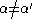. However, it is easy to convert one to the other, using.

The transmission (or transmissivity) is expressed in terms of an absorbance which, for liquids, is defined as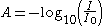whereas, for gases, it is usually defined as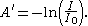This implies that the absorbance becomes linear with the concentration (or number density of absorbers) according to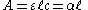and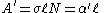for the two cases, respectively.

Thus, if the path length and the molar absorptivity
Molar absorptivity
The molar absorption coefficient, molar extinction coefficient, or molar absorptivity, is a measurement of how strongly a chemical species absorbs light at a given wavelength...

(or the absorption cross section
Absorption cross section
Absorption cross section is a measure for the probability of an absorption process. More generally, the term cross section is used in physics to quantify the probability of a certain particle-particle interaction, e.g., scattering, electromagnetic absorption, etc...

) are known and the absorbance is measured, the concentration of the substance (or the number density of absorbers) can be deduced.

Although several of the expressions above often are used as Beer–Lambert law, the name should strictly speaking only be associated with the latter two. The reason is that historically, the Lambert law states that absorption is proportional to the light path length, whereas the Beer law states that absorption is proportional to the concentration of absorbing species in the material.

If the concentration is expressed as a mole fraction i.e., a dimensionless fraction, the molar absorptivity (ε) takes the same dimension as the absorption coefficient, i.e., reciprocal length
Reciprocal length
Reciprocal length or inverse length is a measurement used in several branches of science and mathematics. As the reciprocal of length, common units used for this measurement include the reciprocal metre or inverse metre , the reciprocal centimetre or inverse centimetre , and, in optics, the...

(e.g., m−1). However, if the concentration is expressed in moles
Mole (unit)
The mole is a unit of measurement used in chemistry to express amounts of a chemical substance, defined as an amount of a substance that contains as many elementary entities as there are atoms in 12 grams of pure carbon-12 , the isotope of carbon with atomic weight 12. This corresponds to a value...

per unit volume
Volume
Volume is the quantity of three-dimensional space enclosed by some closed boundary, for example, the space that a substance or shape occupies or contains....

, the molar absorptivity (ε) is used in L
Litér

·mol−1·cm−1, or sometimes in converted SI units of m2·mol−1.

The absorption coefficient α' is one of many ways to describe the absorption of electromagnetic waves. For the others, and their interrelationships, see the article: Mathematical descriptions of opacity
Mathematical descriptions of opacity
When an electromagnetic wave travels through a medium in which it gets absorbed , it undergoes exponential decay as described by the Beer–Lambert law. However, there are many possible ways to characterize the wave and how quickly it is absorbed...

. For example, α' can be expressed in terms of the imaginary part of the refractive index
Refractive index
In optics the refractive index or index of refraction of a substance or medium is a measure of the speed of light in that medium. It is expressed as a ratio of the speed of light in vacuum relative to that in the considered medium....

, κ, and the wavelength
Wavelength
In physics, the wavelength of a sinusoidal wave is the spatial period of the wave—the distance over which the wave's shape repeats.It is usually determined by considering the distance between consecutive corresponding points of the same phase, such as crests, troughs, or zero crossings, and is a...

of the light (in free space), λ0, according to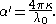In molecular absorption spectrometry, the absorption cross section σ is expressed in terms of a linestrength, S, and an (area-normalized) lineshape function, Φ. The frequency scale in molecular spectroscopy is often in cm−1, wherefore the lineshape function is expressed in units of 1/cm−1, which can look funny but is strictly correct. Since N is given as a number density in units of 1/cm3, the linestrength is often given in units of cm2cm−1/molecule. A typical linestrength in one of the vibrational overtone bands of smaller molecules, e.g., around 1.5 μm in CO or CO2, is around 10−23 cm2cm−1, although it can be larger for species with strong transitions, e.g., C2H2. The linestrengths of various transitions can be found in large databases, e.g., HITRAN. The lineshape function often takes a value around a few 1/cm−1, up to around 10/cm−1 under low pressure conditions, when the transition is Doppler broadened, and below this under atmospheric pressure conditions, when the transition is collision broadened. It has also become commonplace to express the linestrength in units of cm−2/atm since then the concentration is given in terms of a pressure in units of atm. A typical linestrength is then often in the order of 10−3 cm−2/atm. Under these conditions, the detectability of a given technique is often quoted in terms of ppm•m.

The fact that there are two commensurate definitions of absorbance (in base 10 or e) implies that the absorbance and the absorption coefficient for the cases with gases, A' and α', are ln 10 (approximately 2.3) times as large as the corresponding values for liquids, i.e., A and α, respectively. Therefore, care must be taken when interpreting data that the correct form of the law is used.

The law tends to break down at very high concentrations, especially if the material is highly scattering
Scattering
Scattering is a general physical process where some forms of radiation, such as light, sound, or moving particles, are forced to deviate from a straight trajectory by one or more localized non-uniformities in the medium through which they pass. In conventional use, this also includes deviation of...

. If the light is especially intense, nonlinear optical
Nonlinear optics
Nonlinear optics is the branch of optics that describes the behavior of light in nonlinear media, that is, media in which the dielectric polarization P responds nonlinearly to the electric field E of the light...

processes can also cause variances.

## Derivation

The derivation is quite simple in concept. There are many details, so think of this first paragraph as a conceptual overview. Divide the absorbing sample into thin slices that are perpendicular to the beam of light. The light that emerges from a slice is slightly less intense than the light that entered because some of the photons have run into molecules in the sample and did not make it to the other side. For most cases where measurements of absorption are needed, a vast majority of the light entering the slice leaves without being absorbed. Because the physical description of the problem is in terms of differences—intensity before and after light passes through the slice—we can easily write an ordinary differential equation model for absorption. The difference in intensity due to the slice of absorbing material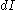is reduced; leaving the slice, it is a fraction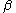of the light entering the slice. The thickness of the slice is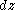, which scales the amount of absorption (thin slice does not absorb much light but a thick slice absorbs a lot). In symbols,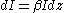, or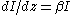. This conceptual overview usesto describe how much light is absorbed. All we can say about the value of this constant is that it will be different for each material. Also, its values should be constrained between −1 and 0. The following paragraphs cover the meaning of this constant and the whole derivation in much greater detail.

Assume that particles may be described as having an absorption cross section (i.e., area), σ, perpendicular to the path of light through a solution, such that a photon of light is absorbed if it strikes the particle, and is transmitted if it does not.

Define z as an axis parallel to the direction that photons of light are moving, and A and dz as the area and thickness (along the z axis) of a 3-dimensional slab of space through which light is passing.
We assume that dz is sufficiently small that one particle in the slab cannot obscure another particle in the slab when viewed along the z direction. The concentration of particles in the slab is represented by N.

It follows that the fraction of photons absorbed when passing through this slab is equal to the total opaque area of the particles in the slab, σAN dz, divided by the area of the slab A, which yields σN dz. Expressing the number of photons absorbed by the slab as dIz, and the total number of photons incident on the slab as Iz, the fraction of photons absorbed by the slab is given by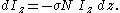Note that because there are fewer photons which pass through the slab than are incident on it, dIz is actually negative (It is proportional in magnitude to the number of photons absorbed).

The solution to this simple differential equation is obtained by integrating both sides to obtain Iz as a function of z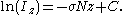The difference of intensity for a slab of real thickness ℓ is I0 at z = 0, and Il at z = ℓ. Using the previous equation, the difference in intensity can be written as,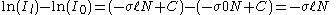rearranging and exponentiating yields,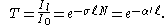This implies that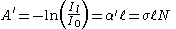and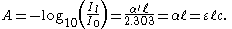The derivation assumes that every absorbing particle behaves independently with respect to the light and is not affected by other particles. Error is introduced when particles are lying along the same optical path such that some particles are in the shadow of others. This occurs in highly concentrated solutions. In practice, when large absorption values are measured, dilution is required to achieve accurate results. Measurements of absorption in the range of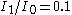to 1 are less affected by shadowing than other sources of random error. In this range, the ODE model developed above is a good approximation; measurements of absorption in this range are linearly related to concentration. At higher absorbances, concentrations will be underestimated due to this shadow effect unless one employs a more sophisticated model that describes the non-linear relationship between absorption and concentration.

## Prerequisites

There are at least six conditions that need to be fulfilled in order for Beer’s law to be valid. These are:
1. The absorbers must act independently of each other;
2. The absorbing medium must be homogeneous in the interaction volume
3. The absorbing medium must not scatter the radiation - no turbidity
Turbidity
Turbidity is the cloudiness or haziness of a fluid caused by individual particles that are generally invisible to the naked eye, similar to smoke in air. The measurement of turbidity is a key test of water quality....

;
4. The incident radiation must consist of parallel rays, each traversing the same length in the absorbing medium;
5. The incident radiation should preferably be monochromatic, or have at least a width that is narrower than that of the absorbing transition; and
6. The incident flux must not influence the atoms or molecules; it should only act as a non-invasive probe of the species under study. In particular, this implies that the light should not cause optical saturation or optical pumping, since such effects will deplete the lower level and possibly give rise to stimulated emission.

If any of these conditions are not fulfilled, there will be deviations from Beer’s law.

## Chemical analysis

Beer's law can be applied to the analysis of a mixture by spectrophotometry, without the need for extensive pre-processing of the sample. An example is the determination of bilirubin
Bilirubin
Bilirubin is the yellow breakdown product of normal heme catabolism. Heme is found in hemoglobin, a principal component of red blood cells. Bilirubin is excreted in bile and urine, and elevated levels may indicate certain diseases...

in blood plasma samples. The spectrum of pure bilirubin is known, so the molar absorbance is known. Measurements are made at one wavelength that is nearly unique for bilirubin and at a second wavelength in order to correct for possible interferences.The concentration is given by c = Acorrected / ε.

For a more complicated example, consider a mixture in solution containing two components at concentrations c1 and c2. The absorbance at any wavelength, λ is, for unit path length, given by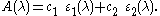Therefore, measurements at two wavelengths yields two equations in two unknowns and will suffice to determine the concentrations c1 and c2 as long as the molar absorbances of the two components, ε1 and ε2 are known at both wavelengths. This two system equation can be solved using Cramer's rule
Cramer's rule
In linear algebra, Cramer's rule is a theorem, which gives an expression for the solution of a system of linear equations with as many equations as unknowns, valid in those cases where there is a unique solution...

. In practice it is better to use linear least squares
Linear least squares
In statistics and mathematics, linear least squares is an approach to fitting a mathematical or statistical model to data in cases where the idealized value provided by the model for any data point is expressed linearly in terms of the unknown parameters of the model...

to determine the two concentrations from measurements made at more than two wavelengths. Mixtures containing more than two components can be analysed in the same way, using a minimum of n wavelengths for a mixture containing n components.

The law is used widely in infra-red spectroscopy for analysis of polymer degradation
Polymer degradation is a change in the properties—tensile strength, colour, shape, etc.—of a polymer or polymer-based product under the influence of one or more environmental factors such as heat, light or chemicals such as acids, alkalis and some salts...

and oxidation. The carbonyl group absorption at about 6 micrometres can be detected quite easily, and degree of oxidation of the polymer
Polymer
A polymer is a large molecule composed of repeating structural units. These subunits are typically connected by covalent chemical bonds...

calculated.

## Beer–Lambert law in the atmosphere

This law is also applied to describe the attenuation of solar or stellar radiation as it travels through the atmosphere. In this case, there is scattering of radiation as well as absorption. The Beer–Lambert law for the atmosphere is usually written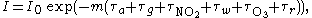where each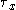is the optical depth
Optical depth
Optical depth, or optical thickness, is a measure of transparency. Optical depth is defined by the negative logarithm of the fraction of radiation that is not scattered or absorbed on a path...

whose subscript identifies the source of the absorption or scattering it describes:
•refers to aerosols (that absorb and scatter)
•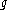are uniformly mixed gases (mainly carbon dioxide
Carbon dioxide
Carbon dioxide is a naturally occurring chemical compound composed of two oxygen atoms covalently bonded to a single carbon atom...

(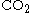) and molecular oxygen
Oxygen
Oxygen is the element with atomic number 8 and represented by the symbol O. Its name derives from the Greek roots ὀξύς and -γενής , because at the time of naming, it was mistakenly thought that all acids required oxygen in their composition...

(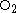) which only absorb)
•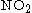is nitrogen dioxide
Nitrogen dioxide
Nitrogen dioxide is the chemical compound with the formula it is one of several nitrogen oxides. is an intermediate in the industrial synthesis of nitric acid, millions of tons of which are produced each year. This reddish-brown toxic gas has a characteristic sharp, biting odor and is a prominent...

, mainly due to urban pollution (absorption only)
•is water vapour absorption
Water absorption
During the transmission of electromagnetic radiation through a medium containing water molecules, portions of the electromagnetic spectrum are absorbed by water molecules...

•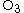is ozone
Ozone
Ozone , or trioxygen, is a triatomic molecule, consisting of three oxygen atoms. It is an allotrope of oxygen that is much less stable than the diatomic allotrope...

(absorption only)
•is Rayleigh scattering
Rayleigh scattering
Rayleigh scattering, named after the British physicist Lord Rayleigh, is the elastic scattering of light or other electromagnetic radiation by particles much smaller than the wavelength of the light. The particles may be individual atoms or molecules. It can occur when light travels through...

from molecular oxygen
Oxygen
Oxygen is the element with atomic number 8 and represented by the symbol O. Its name derives from the Greek roots ὀξύς and -γενής , because at the time of naming, it was mistakenly thought that all acids required oxygen in their composition...

() and nitrogen
Nitrogen
Nitrogen is a chemical element that has the symbol N, atomic number of 7 and atomic mass 14.00674 u. Elemental nitrogen is a colorless, odorless, tasteless, and mostly inert diatomic gas at standard conditions, constituting 78.08% by volume of Earth's atmosphere...

(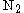) (responsible for the blue color of the sky).is the optical mass or airmass factor
Airmass
In astronomy, air mass is the optical path length through Earth’s atmosphere for light from a celestial source. As it passes through the atmosphere, light is attenuated by scattering and absorption; the more atmosphere through which it passes, the greater the attenuation. Consequently, celestial...

, a term approximately equal (for small and moderate values of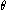) to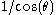, whereis the observed object's zenith angle
Celestial coordinate system
In astronomy, a celestial coordinate system is a coordinate system for mapping positions on the celestial sphere.There are different celestial coordinate systems each using a system of spherical coordinates projected on the celestial sphere, in analogy to the geographic coordinate system used on...

(the angle measured from
the direction perpendicular to the Earth's surface at the observation site).

This equation can be used to retrieve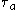, the aerosol optical thickness
Optical depth
Optical depth, or optical thickness, is a measure of transparency. Optical depth is defined by the negative logarithm of the fraction of radiation that is not scattered or absorbed on a path...

,
which is necessary for the correction of satellite images and also important in accounting for the role of
aerosols in climate.

When the path taken by the light is through the atmosphere, the density of the absorbing gas is not constant, so the original equation must be modified as follows: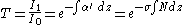where z is the distance along the path through the atmosphere, all other symbols are as defined above. This is taken into account in eachin the atmospheric equation above.

## History

The law was discovered by Pierre Bouguer
Pierre Bouguer
Pierre Bouguer was a French mathematician, geophysicist, geodesist, and astronomer. He is also known as "the father of naval architecture"....

before 1729. It is often attributed to Johann Heinrich Lambert
Johann Heinrich Lambert
Johann Heinrich Lambert was a Swiss mathematician, physicist, philosopher and astronomer.Asteroid 187 Lamberta was named in his honour.-Biography:...

, who cited Bouguer's Essai d'Optique sur la Gradation de la Lumiere (Claude Jombert, Paris, 1729) — and even quoted from it — in his Photometria in 1760. Much later, August Beer
August Beer
August Beer was a German physicist and mathematician. Beer was born in Trier, where he studied mathematics and natural sciences. He worked for Julius Plücker in Bonn afterwards, where he earned a Ph.D. in 1848 and became a lecturer in 1850. In 1854, Beer published his book Einleitung in die höhere...

extended the exponential absorption law in 1852 to include the concentration of solutions in the absorption coefficient.

• Applied spectroscopy
Applied spectroscopy
Applied spectroscopy is the application of various spectroscopic methods for detection and identification of different elements/compounds in solving problems in the fields of forensics, medicine, oil industry, atmospheric chemistry, pharmacology, etc....

• Atomic absorption spectroscopy
Atomic absorption spectroscopy
Atomic absorption spectroscopy is a spectroanalytical procedure for the qualitative and quantitative determination of chemical elements employing the absorption of optical radiation by free atoms in the gaseous state. In analytical chemistry the technique is used for determining the concentration...

• Absorption spectroscopy
Absorption spectroscopy
Absorption spectroscopy refers to spectroscopic techniques that measure the absorption of radiation, as a function of frequency or wavelength, due to its interaction with a sample. The sample absorbs energy, i.e., photons, from the radiating field. The intensity of the absorption varies as a...

• Cavity ring-down spectroscopy
• Infra-red spectroscopy
• Job plot
Job plot
A Job plot is used to determine the stoichiometry of a binding event. This method is widely used in analytical chemistry, instrumental analysis, and advanced chemical equilibrium texts and research articles.In solutions where two species are present A Job plot (also known as the method of...

• Laser absorption spectrometry
Laser absorption spectrometry
Laser absorption spectrometry refers to techniques that use lasers to assess the concentration or amount of a species in gas phase by absorption spectrometry ....

• Logarithm
Logarithm
The logarithm of a number is the exponent by which another fixed value, the base, has to be raised to produce that number. For example, the logarithm of 1000 to base 10 is 3, because 1000 is 10 to the power 3: More generally, if x = by, then y is the logarithm of x to base b, and is written...

Polymer degradation is a change in the properties—tensile strength, colour, shape, etc.—of a polymer or polymer-based product under the influence of one or more environmental factors such as heat, light or chemicals such as acids, alkalis and some salts...

• Scientific laws named after people
Scientific laws named after people
This is a list of scientific laws named after people . For other lists of eponyms, see eponym.-See also:* Eponym* Fields of science...

• Quantification of nucleic acids
Quantification of nucleic acids
In molecular biology, quantitation of nucleic acids is commonly performed to determine the average concentrations of DNA or RNA present in a mixture, as well as their purity. Reactions that use nucleic acids often require particular amounts and purity for optimum performance...

• Tunable diode laser absorption spectroscopy